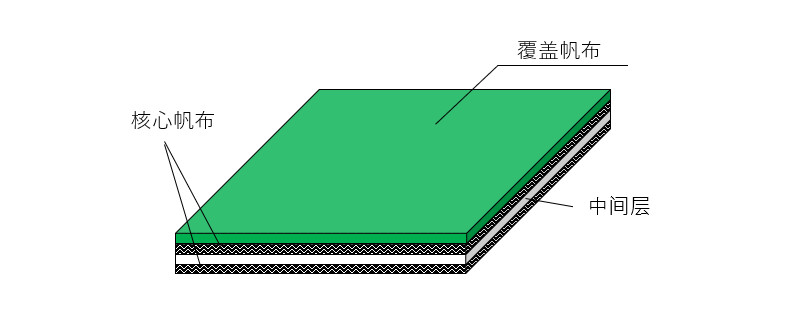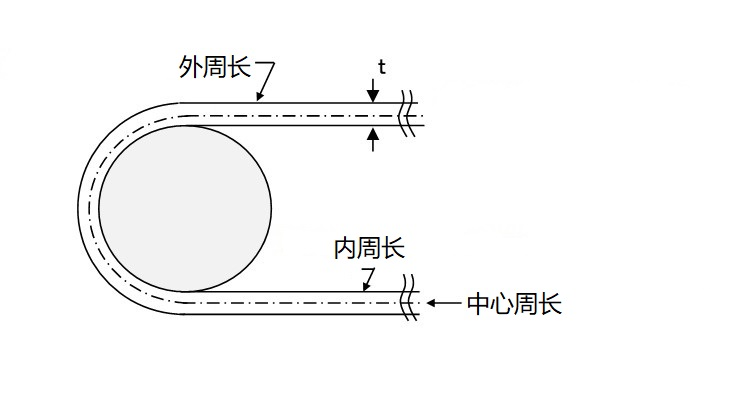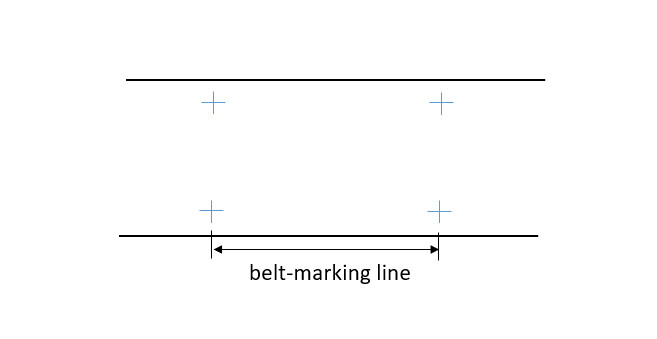1. 三之星机带株式会社
2. 支持/下载
3. 常见问题

## 常见问题

### 汽车和摩托车用传动带及工程机械和工业机械用传动机带

Q

A

Q

A

Q

A

Q

A

Q

A

Star Fit™是特殊的V形多楔带，用于皮带轮不移动的特定发动机。它由不同于普通V形肋带的材料制成，可以在不移动滑轮的情况下安装，同时保持适当的张力。

Q

A

Q

A

### 一般产业用传动带及相关产品

Q

V型带是否符合RoHS2标准？

A

Q

A

Q

V型带的初始伸长率是多少？

A

Q

V型带的材料是什么？

A

Q

A

Q

A

V形带的长度各不相同。如果你担心长度上的差异，请指定为匹配的套装。

Q

A

Q

A

【橡胶带】齿形：氯丁橡胶 拉伸绳：玻璃代码 齿形织物：尼龙
【聚氨酯带】齿形：聚氨酯 拉伸绳：芳纶或钢丝

Q

A

Q

A

Q

A

Q

A

Q

A

Q

A

※重新张紧同步带可能导致啮合不良并缩短皮带的寿命。

Q

A

Q

A

Q

A

Q

A

Q

A

Q

A

Q

A

Q

A

※美高特GⅡP、美高特GⅢ和极高特GX不能用挠性负载测量。

Q

A

Q

A

Q

A

Q

A

Q

A

Q

A

Q

A

Q

A

Q

A

Q

A

Q

A

※有些尺寸不被支持。

Q

A

Q

A

Q

A

Q

A

min=最小的轴孔直径
max=最大的轴孔直径

Q

A

Q

A

Q

A

Q

A

Q

A

Q

A

Q

A

Q

A

Q

A

Q

A

Q

A/B/C型V带和LA/LB/LC型V带之间有什么区别？

A

LA/LB/LC型是用于农业机械的皮带。这些皮带比标准V型皮带和红标V型皮带（A/B/C型）更薄，并被设计成可以承受反向弯曲。

Q

A

Q

A

### 输送带及系统相关产品

Q

A

□输送带的结构和名称(Tailorbelt™)Q

A【図 1．输送带的长度】

Q

A

Q

A

Tailorbelt®-U,V,P：皮带伸长率为0.1-0.3%。
Tailorbelt®-F(FG type）：皮带伸长率为0.05-0.1%
Tailorbelt®-F(FK type）：皮带伸长率为0.1-0.2%

Q

A【図 3．带状标记线】

※避免靠近接头和标记线的区域。
※当皮带宽度较宽时，如上图所示，在左边和右边划线。
➡在Tailorbelt™-U的情况下
初始张力（约）0.1-0.3%在皮带伸长率。
对于1000毫米的标记，施加张力使其为1至3毫米。
※标记线应该在一个平坦的地方测量，没有滑轮或类似东西造成的弯折。
※张力应平均施加在左右两侧。

### 合成树脂材料及加工品

Q

A

Q

A

Q

A

Q

A

Q

A

Q

A

Q

A

MC尼龙 "是三菱化学先进材料公司商标下的一个广为人知的商品名称。

Q

A

6个尼龙。 浇铸尼龙是通过将原材料倒入模具并进行聚合而浇铸成型的，与挤出的6-尼龙相比，它的机械强度更高，吸水率更低。

Q

POM均聚物和共聚物的区别是什么？

A

Q

A

### 电子材料、涂料

Q

A

Q

A

Q

A

Q

A

Q

A

Q

A

Q

A

Q

A

Q

A

Q

A

Q

A

Q

A

Q

A

Q

A

Q

A

Q

A

Q

A

Q

A

Q

A

Q

A

Q

A

Q

A

Q

A

Q

A

Q

A

Q

A

Q

A

Q

A

Q

A

Q

A

Q

A

Q

A

Q

A

Q

A

Q

A

Q

A

Q

A

Q

A

Q

A

Q

A

Q

A

Q

A

Q

A

Q

A

Q

A

Q

A

#### 热压机缓冲垫

Q

1层、2层和3层之间的区别是什么？

A

Q

A

Q

M系列和D系列之间有什么区别？

A

Q

A

Q

A

Q

A

Q

A

Q

A

Q

A

Q

A

Q

A

Q

A

Q

A

60wt%或以下。调整也是可能的

Q

A

Q

A

Q

A